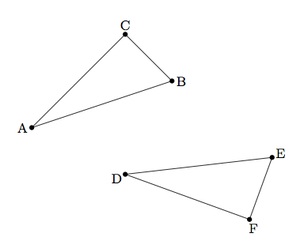# SSS Congruence Criterion

Alignments to Content Standards: G-CO.B.8

Suppose $\triangle ABC$ and $\triangle DEF$ share three corresponding congruent sides as pictured below:Show that $\triangle ABC$ is congruent ot $\triangle DEF$ as follows:

1. Apply a translation to move $\triangle ABC$ to $\triangle A^\prime B^\prime C^\prime$ with $A^\prime = D$.
2. Apply a rotation to move $\triangle A^\prime B^\prime C^\prime$ to $A^{\prime \prime}B^{\prime \prime}C^{\prime \prime}$ with $A^{\prime \prime} = D$ and $B^{\prime \prime} = E$.
3. Explain why $\left|A^{\prime \prime}C^{\prime \prime}\right| = \left|DF\right|$ and conclude that $\overleftrightarrow{DE}$ is the perpendicular bisector of $\overline{C^{\prime \prime}F}$.
4. Show that reflection over $\overleftrightarrow{DE}$ maps $F$ to $C^{\prime \prime}$ and conclude that $\triangle ABC$ is congruent to $\triangle DEF$.

## IM Commentary

The goal of this task is to establish the SSS congruence criterion using rigid motions. The method used here relies on understanding that the perpendicular bisector of a segment $|PQ|$ is the set of points in the plane equidistant from $P$ and $Q$: see https://www.illustrativemathematics.org/illustrations/967. The argument presented here treats a specific triangle congruence but the steps outlined here can be adapted to show the congruence of any two triangles sharing three corresponding congruent sides:

• The translation is not necessary when $A = D$.
• The rotation is not necessary if $B^\prime = E$ (or if $A$ = $D$ and $B$ = $E$).
• The reflection is not necessary if $C^{\prime \prime}$ lies on the same side of $\overleftrightarrow{DE}$ as $F$.

Note, however, that in the case where no reflection is required, a further argument is needed to show the triangle congruence. One method which can be used is to reflect over $\overleftrightarrow{DE}$ and then apply the argument in the solution.

It is useful for teachers and students to compare the SSS congruence criterion to the SAS congruence criterion (see https://www.illustrativemathematics.org/illustrations/109). The SSS congruence criterion is fundamentally more difficult to prove than the SAS criterion. Another proof of the SSS criterion is presented in https://www.illustrativemathematics.org/illustrations/110 and it uses the SAS congruence criterion.

## Solution

1. The image of $\triangle ABC$ after translation by $\overline{AD}$ is pictured below, labeled $A^\prime B^\prime C^\prime$:The translation is chosen to send $A$ to $D$ so $A^\prime = D$ in the picture. Since $\triangle A^\prime B^\prime C^\prime$ is a translate of $\triangle ABC$ they are congruent and corresponding sides are congruent since translations preserve distance.

2. If we rotate $\triangle A^\prime B^\prime C^\prime$ about  $A^\prime$ by $\angle B^\prime DE$ then $A^\prime = D$ does not move and $B^\prime$ maps to $E$. The image of $\triangle A^\prime B^\prime C^\prime$ after this rotation is shown below, labeled $\triangle A^{\prime \prime}B^{\prime \prime}C^{\prime \prime}$:Since $\triangle A^{\prime \prime} B^{\prime \prime} C^{\prime \prime}$ is a rotation of $\triangle A^\prime B^\prime C^\prime$ they are congruent and $\triangle A^{\prime \prime} B^{\prime \prime} C^{\prime \prime}$ is also congruent to $\triangle ABC$. Corresponding sides of these triangles are congruent since rotations (and translations) preserve distance.

3. We know that $|AC| = |DF|$ by hypothesis. We saw in parts (a) and (b) that $\left|A^{\prime \prime} C^{\prime \prime} \right| = |AC|$ so we have $\left|A^{\prime \prime} C^{\prime \prime} \right| = |DF|$. Similar reasoning shows that $\left|B^{\prime \prime}C^{\prime \prime}\right| = |EF|$. The perpendicular bisector of $\overline{C^{\prime \prime}F}$ is the set of points equidistant from $C^{\prime \prime}$ and $F$ so this means that $D$ and $E$ both lie on the perpendicular bisector of $\overline{C^{\prime \prime}F}$. Thus $\overleftrightarrow{DE}$ is the perpendicular bisector of $\overline{C^{\prime \prime}F}$.
4. We saw in part (c) that $\overleftrightarrow{DE}$ is the perpendicular bisector of $\overline{C^{\prime \prime}F}$. This means that reflection over $\overleftrightarrow{DE}$ maps $F$ to $C^{\prime \prime}$. This reflection maps $D$ and $E$ to themselves and so maps $\triangle A^{\prime \prime}B^{\prime \prime}C^{\prime \prime}$ to $\triangle DEF$, establishing the congruence of $\triangle ABC$ with $\triangle DEF$.